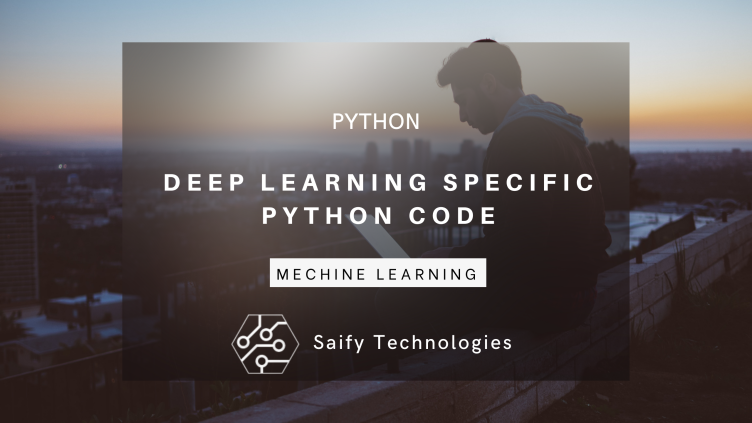# DEEP LEARNING SPECIFIC PYTHON CODE

Python is a programming language written by Guido Van Rossum for making programming easy and efficient. It was learn and easy to maintain. It is adopted by scientific community because of it ease of use. In the beginning python was not object oriented language but as its popularity grows it is converted into objects oriented language.

For learning deep learning we need to learn python as all of the deep learning framework are written in python, and python has a huge eco system. It contains huge libraries to support you in deep learning. Libraries such as numpy, pandas etc are the backbone of deep learning and secondly installation of libraries are also too easy with pip manager. Python is easy to learn for both new comers and already to the people who know programming. For new comers he don't have to learn messy symbols alignment and for ready mode programmers he just need to understand use of indentation (use of space in right places)

Python has achieved its popularity that much that all big companies uses python for its research and production of application. Google, Apple, Microsoft all of this big IT giants uses python in some or other way. Python has a huge community to help you out if you stuck in some problem. We learn python because all deep learning framework are written in python such as Tensorflow, Pytarch, CNTK, Mxnet and many more to come.

We will discuss all basic required function and concept needed for deep learning in Python.

### VARIABLE

It is a placeholder of certain amount or numerical or string value in program. In other language variable is typed input means it has some datatypes but in python. It is just a variable python compiler will take care of datatype by seeing its value. We directly assign variable in pyhton

`a=7 int a=7`

`a=7.5 float b=7.5`

`a="taher" string c="tahe"`

### CONDITIONAL OPERATORS

It is conditional operator in which we use if and else statement. If statement is use to decide that we have run the given code inside if statement. If statement contains certain condition is fulfilled then only D code below of If statement will be run

Syntax is python for if else statement is

`x=2`
`if x==2`
`print("hello")`
`else`
`print("x is 2")`

Syntax in JAVA

## LOOP IN PYTHON

Loops in programming language is used to run code multiple times which is present in the loop. We uses FOR and WHILE for running inside loops in python. We ned to provide same expression which is when met then only that loop will executed.

Syntax for FOR and WHILE loop in python

## FUNCTIONS

Function is defined as bunch of lines of code which is used to perform certain function or operation in the program. It is made by the methology so that you write only one time and use that code as many times as you want. Different type of programming language uses different syntax and you need to provide return data type and input datatype in a function. But in python we don't need to define its input and return datatype because it is maintain by python computer.

Example and Syntax in python we will make addition function of inputs and return its output.

`a=5`
`b=11`
`def addition(c,d):`
`e=c+d`
`return e`
`print(addition(a,b))`

Here above we have defined two variable a and b with value 5 and 11 and also defined addition function where we have defined that c and d as this function will get any two variable and this function have to add this two variable and return this value and at the end we print addition function value.

## LAMBDA FUNCTION

A lambda function is a small anonymous function used to perform some operation. A lambda function can take any number of arguments, but can only have one expression.

Here above we have defined two variable a and b with value 5 and 11 and also defined addition function where we have defined that c and d as this function will get any two variable and this function have to add this two variable and return this value and at the end we print addition function value.

## LAMBDA FUNCTION

A lambda function is a small anonymous function used to perform some operation. A lambda function can take any number of arguments, but can only have one expression.

`x=lambdaa:a+10`
`print(x(5))`

The power of lambda function is used when it is used inside function

`def myfunc(x):`
`return lambda a:a*n`
`mydoubler=myfunc(2)`
`print(mydoubler(11))`

When declare first time myfunc lambda a is set and we use mydoubler second time then x is set and output is carried as 22

## CONS

Cons of using python is that it is not safe and fast either and use much more memory than needed. Compare to other programming language. Python is developed to make programming easy in the cost of speed.

## CONCLUSION

In this post we have explore some of the basic python function needed to learn deep learning efficiently.

Code Published on :01/08/2022